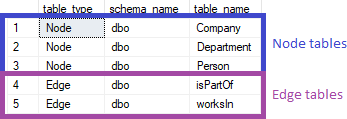## List graph tables in Azure SQL database

Article for:

Query below returns graph tables.

## Query

``````select case when is_node = 1 then 'Node'
when is_edge = 1 then 'Edge'
end table_type,
schema_name(schema_id) as schema_name,
name as table_name
from sys.tables
where is_node = 1 or is_edge = 1
order by is_edge, schema_name, table_name
``````

## Columns

• table_type - graph table type
• Node
• Edge
• schema_name - table schema name
• table_name - table name

## Rows

• One row represents one graph table - node or edge
• Scope of rows: only graph (node or edge) tables are included
• Ordered by nodes first, then edeges. Within type by schema and table name

## Sample results